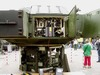1958

X(k)=A X(k-1)+B U(k)+W(k)

KALMAN FILTER

• 扩展卡尔曼滤波（Extended Kalman Filter，EKF）是标准卡尔曼滤波在非线性情形下的一种扩展形式，它是一种高效率的递归滤波器（自回归滤波器）。 EKF的基本思想是利用泰勒级数展开将非线性系统线性化，然后采用...
更新日志：

2020.02.13：修改了第三节推导中的公式错误

2020.03.21：修改了2.1节中的部分表述和公式加粗，补充迹的求导公式

1 简介

扩展卡尔曼滤波（Extended Kalman Filter，EKF）是标准卡尔曼滤波在非线性情形下的一种扩展形式，它是一种高效率的递归滤波器（自回归滤波器）。

EKF的基本思想是利用泰勒级数展开将非线性系统线性化，然后采用卡尔曼滤波框架对信号进行滤波，因此它是一种次优滤波。

2 算法介绍

2.1 泰勒级数展开

泰勒级数展开是将一个在处具有阶导数的函数，利用关于的次多项式逼近函数值的方法。

若函数在包含的某个闭区间上具有阶导数，且在开区间上具有阶导数，则对闭区间上的任意一点，都有：

其中表示函数在处的阶导数，等式右边成为泰勒展开式，剩余的是泰勒展开式的余项，是的高阶无穷小。

（著名的欧拉公式就是利用，和的泰勒展开式得来的！）

当变量是多维向量时，一维的泰勒展开就需要做拓展，具体形式如下：

其中，表示雅克比矩阵，表示海塞矩阵，表示高阶无穷小。

这里，为维，状态向量为维，。

一般来说，EKF在对非线性函数做泰勒展开时，只取到一阶导和二阶导，而由于二阶导的计算复杂性，更多的实际应用只取到一阶导，同样也能有较好的结果。取一阶导时，状态转移方程和观测方程就近似为线性方程，高斯分布的变量经过线性变换之后仍然是高斯分布，这样就能够延用标准卡尔曼滤波的框架。

2.1 EKF

标准卡尔曼滤波KF的状态转移方程和观测方程为

扩展卡尔曼滤波EKF的状态转移方程和观测方程为

（1）

（2）

利用泰勒展开式对（1）式在上一次的估计值处展开得

（3）

再利用泰勒展开式对（2）式在本轮的状态预测值处展开得

（4）

其中，和分别表示函数和在和处的雅克比矩阵。

（注：这里对泰勒展开式只保留到一阶导，二阶导数以上的都舍去，噪声假设均为加性高斯噪声）

基于以上的公式，给出EKF的预测（Predict）和更新（Update）两个步骤：

Propagation：

Update：

其中的雅克比矩阵和分别为

，

雅可比矩阵的计算，在MATLAB中可以利用对自变量加上一个eps（极小数），然后用因变量的变化量去除以eps即可得到雅可比矩阵的每一个元素值。

读者可能好奇？为什么扩展卡尔曼滤波EKF的传播和更新的形式会和标准卡尔曼滤波KF的形式一致呢？以下做一个简单的推导。

3 推导

先列出几个变量的表示、状态转移方程和观测方程：

真实值，预测值，估计值，观测值，观测值的预测，估计值与真实值之间的误差协方差矩阵，求期望的符号。

，

，

引入反馈：      （5）

OK，可以开始推导了：

由公式（3）（4）得到以下两个等式，标为式（6）（7）

计算估计值与真实值之间的误差协方差矩阵，并把式子（4）（5）（7）代入，得到

其中表示真实值与与预测值之间的误差协方差矩阵。于是得到式（8）

因为的对角元即为真实值与估计值的误差的平方，矩阵的迹（用表示）即为总误差的平方和，即

利用以下矩阵迹的求导公式（其中和表示矩阵，表示列向量）：

要让估计值更接近于真实值，就要使上面的迹尽可能的小，因此要取得合适的卡尔曼增益，使得迹得到最小，言外之意就是使得迹对的偏导为0，即

这样就能算出合适的卡尔曼增益了，即

代回式（8）得到

接下来就差真实值与预测值之间的协方差矩阵的求值公式了

将以下两个等式代入到上式，

，

可以得到

有、与观测噪声是独立的，求期望等于零;；表示观测噪声的协方差矩阵，用表示。于是得到

其中的协方差矩阵的转置矩阵就是它本身。OK，大功告成，以上就完成了全部公式的推导了。

4 实际应用

现在我们假设在海上有一艘正在行驶的船只，其相对于传感器的横纵坐标为为隐藏状态，无法直接获得，而传感器可以测量得到船只相对于传感器的距离和角度，传感器采样的时间间隔为，则：

状态向量，观测向量

状态转移方程和观测方程为：

那么雅克比矩阵为

这里给定距离传感器的噪声均值为，方差为；角度传感器的噪声均值为0，方差为（单位弧度）；

采样时间点为个；

船只的初始状态为，四个状态量的噪声的方差分别为。仿真结果如下：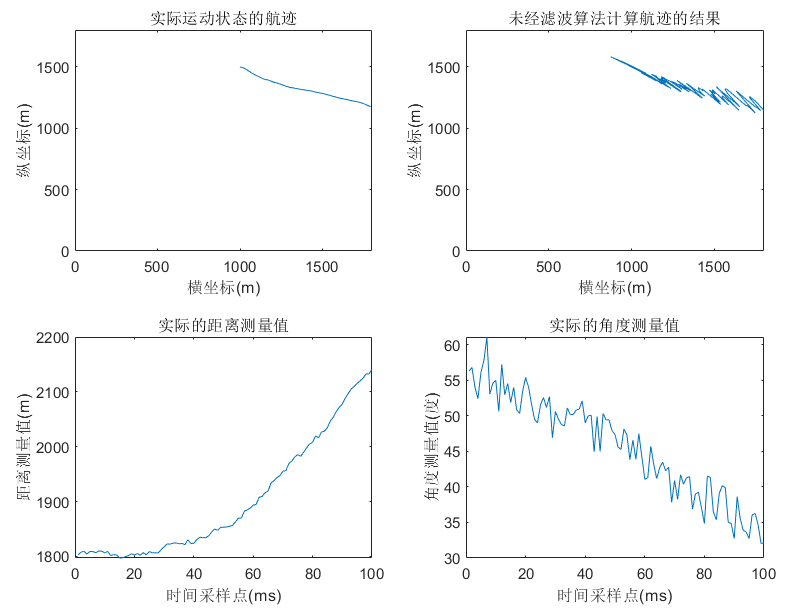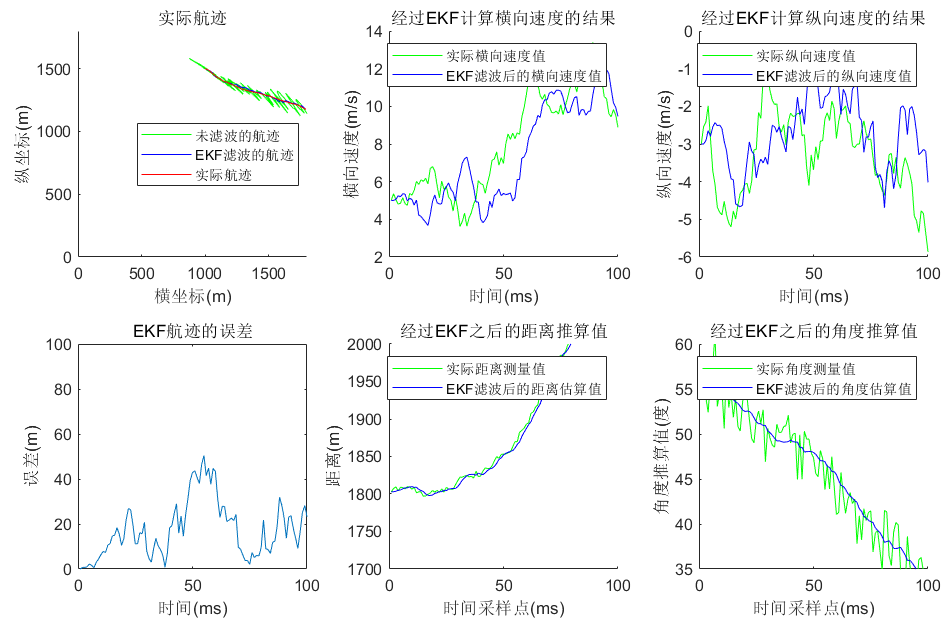从仿真结果可以看出，EKF在这种情形下的滤波效果还是不错的，但是在实际应用中，对于船只运动的状态转移噪声的均值和协方差矩阵，以及传感器的观测噪声的均值和协方差矩阵，往往都是未知的，有很多情况都只有观测值而已，这样的情形下，就有必要利用观测值对噪声的统计量参数做出适当的估计（学习）。

5 参数估计（参数学习）

，

，

（1）E-Step

Propagation：

Update：

（2）M-Step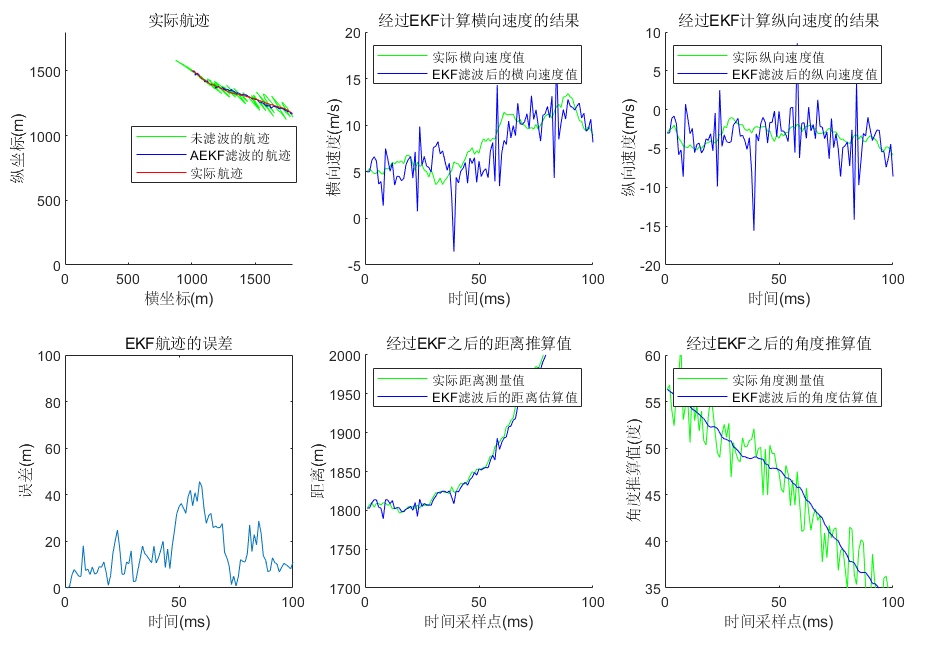6 总结

EKF滤波通过泰勒展开公式，把非线性方程在局部线性化，使得高斯分布的变量在经过线性变换后仍然为高斯分布，这使得能继续把标准卡尔曼滤波KF的框架拿过来用，总体来说，EKF在函数的非线性不是很剧烈的情形下，能够具有很不错的滤波效果。但是EKF也有它的不足之处：其一，它必须求解非线性函数的Jacobi矩阵，对于模型复杂的系统，它比较复杂而且容易出错；其二，引入了线性化误差，对非线性强的系统，容易导致滤波结果下降。基于以上原因，为了提高滤波精度和效率，以满足特殊问题的需要，就必须寻找新的逼近方法，于是便有了粒子滤波PF和无迹卡尔曼滤波UKF，笔者将在接下来的博文中为读者解读。

7 参考文献

 林鸿. 基于EM算法的随机动态系统建模[J]. 福建师大学报(自然科学版), 2011, 27(6):33-37.

 https://www.cnblogs.com/gaoxiang12/p/5560360.html.

 https://max.book118.com/html/2017/0502/103920556.shtm.

原创性声明：本文属于作者原创性文章，小弟码字辛苦，转载还请注明出处。谢谢~

如果有哪些地方表述的不够得体和清晰，有存在的任何问题，亦或者程序存在任何考虑不周和漏洞，欢迎评论和指正，谢谢各路大佬。

需要代码和有需要相关技术支持的可咨询QQ：297461921

展开全文信号处理
• 很有用的卡尔曼滤波算法实例和 扩展卡尔曼滤波算法实例
• 卡尔曼滤波算法及扩展卡尔曼滤波在信号估计中的应用
• 卡尔曼滤波，是当前最优估计理论中十分重要的一个部分。 要全面地理解卡尔曼滤波，你需要一点统计学的知识，以及一点矩阵理论。通常最优估计理论的教材是从最小二乘估计讲起，接着讲到最小方差估计，极大似然估计...
卡尔曼滤波，是最优估计理论中十分重要的一个部分。
要全面地理解卡尔曼滤波，你需要一点统计学的知识，以及一点矩阵理论。通常最优估计理论的教材是从最小二乘估计讲起，接着讲到最小方差估计，极大似然估计以及维纳滤波，再到卡尔曼滤波、扩展卡尔曼、无迹卡尔曼——-这是从统计的角度来理解卡尔曼滤波。
事实上，卡尔曼滤波与《自动控制原理》中的状态观测器非常相似。状态观测器利用原系统的输入输出来估计系统状态，对原系统建模(状态方程，输出方程)，把观测器的输出与原系统输出的差乘上一个增益反馈给观测器的状态，这样构成一个闭环系统使得观测器状态跟踪原系统状态。卡尔曼滤波中，同样要对系统建模得到状态方程，输出方程则取决于你的测量手段，因此改称量测方程。卡尔曼同样要预测量测值，并把预测值与真实量测值比较，乘上一个增益返回给状态。不同之处在于，状态观测器不考虑噪声，要求系统可检测，并且增益通常是时不变的，而卡尔曼滤波则详细考虑了噪声和初始估计误差，智能的计算每一步的增益，以达到对状态的最优的估计。

实际工程常常面临这样的情况：变量X$X$无法直接测量，能测量的是一些与X$X$相关的量Z$Z$，通常可以得到确定的函数关系：Z=H(X)$Z=H(X)$，例如要用mpu6050测量转动欧拉角(ψ,θ,γ)T$(\psi, \theta,\gamma)^T$，无法直接测量，但是加速度计的测量值与欧拉角有关系：
⎡⎣⎢axayaz⎤⎦⎥=⎡⎣⎢−gsinγcosθgsinθgcosγcosθ⎤⎦⎥\begin{bmatrix}a_x\\a_y\\a_z\end{bmatrix}=\begin{bmatrix}-g\sin\gamma \cos\theta\\g\sin\theta\\g\cos\gamma\cos\theta\end{bmatrix}
由测量值Z估计状态X，典型的方法–最小二乘估计，即对X的估计—X^$\hat X$应当使得E{(Z−H(X^))(Z−H(X^))T}$E\{(Z-H(\hat X))(Z-H(\hat X))^T\}$最小。典型例子如用直尺测量一支笔的长度，多次测量取平均值就是一个最简单的最小二乘估计。
实际工程中，对状态X$X$可以通过建模，给出X$X$的动力学过程：X˙=f(X,t)$\dot X=f(X,t)$，若能确定初始时刻的状态X0$X_0$，那么显然初始时刻以后的状态都可以确定，对状态X$X$的确定就是一个微分方程求解的问题。然而实际系统f(X)$f(X)$多具有复杂的形式，给出X$X$的解析解是一件困难甚至不可能的事。好在我们并不要求X$X$绝对精确的值，只需要满足精度需求的值，那么我们可以依靠数值积分方法给出状态X$X$的解，只要步长Δt$\Delta t$取得足够小，龙格库塔法正是其中的一种典型方法。
X$X$的动力学过程与对Z$Z$的测量都包含有关于X$X$的信息，卡尔曼滤波正是综合运用了这两种信息来给出状态x的最优估计。
卡尔曼滤波包含两个基本方程–状态方程和量测方程，这也是卡尔曼滤波最根本的地方，但凡要实现一个卡尔曼滤波器，只要写出状态方程和量测方程，剩下的就只是代公式了，如果连状态方程和测量方程都写不出来搞不清楚，那也别谈什么实现卡尔曼滤波器。
状态方程：Xk=Φk/k−1Xk−1+Γk−1Wk−1X_{k}=\Phi_{k/k-1}X_{k-1}+\Gamma_{k-1}W_{k-1}
状态方程描述了状态X$X$的动力学过程，Φk/k−1$\Phi_{k/k-1}$是从k-1时刻到k时刻的状态转移矩阵，Γk−1$\Gamma_{k-1}$是k-1时刻的噪声输入矩阵，Wk−1$W_{k-1}$是k-1时刻的系统噪声。
量测方程：Zk=HkXk+VkZ_{k}=H_kX_k+V_k
量测方程描述了状态与量测量的关系，Vk$V_k$是k时刻的测量噪声。
上述两个方程是标准线性卡尔曼的基本方程，它要求两个方程是线性的，还要求系统噪声W$W$和测量噪声V$V$都是白噪声。
卡尔曼的推导过程需要较强的数学基础，这里不讨论，只说明卡尔曼的具体做法和物理意义。
卡尔曼要解决的问题：若在k-1时刻估计状态为X^k−1$\hat X_{k-1}$，在k时刻有一个测量Zk$Z_k$，那么k时刻对状态的预测X^k$\hat X_k$应该是多少呢？
回答这个问题，需要考察状态方程：
Xk=Φk/k−1Xk−1+Γk−1Wk−1X_{k}=\Phi_{k/k-1}X_{k-1}+\Gamma_{k-1}W_{k-1}
和量测方程：
Zk=HkXk+VkZ_{k}=H_kX_k+V_k
以及必要的假设：系统噪声W$W$和测量噪声V$V$都是白噪声，即E(Wk)=0$E(W_{k})=0$，E(WkWTj)=Qkδkj$E(W_kW_j^T)=Q_k \delta_{kj}$，E(Vk)=0$E(V_{k})=0$，E(VkVTj)=Rkδkj$E(V_kV_j^T)=R_k \delta_{kj}$，并且假设k-1时刻状态估计为X^k−1$\hat X_{k-1}$，误差协方差为Pk−1=E(Xk−1−X^k−1)(Xk−1−X^k−1)T$P_{k-1}=E(X_{k-1}-\hat X_{k-1})(X_{k-1}-\hat X_{k-1})^T$。
首先，既然在k-1时刻已经估计出状态为X^k−1$\hat X_{k-1}$，那么k时刻的状态按照状态方程就应当预测为： X^k/k−1=Φk/k−1X^k−1\hat X_{k/k-1}=\Phi_{k/k-1} \hat X_{k-1} X^k/k−1$\hat X_{k/k-1}$被称为状态一步预测值。
既然对k时刻状态做出预测，那么显然可以根据量测方程再对k时刻的测量值做出预测：Z^k=HkX^k/k−1\hat Z_k=H_k\hat X_{k/k-1}。
既然对测量做出了预测，又获得了一个真正的测量值Zk$Z_k$，那么显然它们的差Zk−Z^k$Z_k-\hat Z_k$反映了预测状态与真实状态的差。所以毫无疑问，需要利用这个差对状态做出修正：X^k=X^k/k−1+Kk(Zk−HkX^k/k−1)\hat X_k=\hat X_{k/k-1}+K_k(Z_k-H_k\hat X_{k/k-1})
Kk$K_k$是增益阵，那么剩下的问题就是如何选取Kk$K_k$。选取Kk$K_k$的原则是使得误差协方差阵 E(Xk−X^k)(Xk−X^k)T$E(X_k-\hat X_k)(X_k-\hat X_k)^T$最小，按照上式，对Kk$K_k$的选取既要考虑X^k/k−1$\hat X_{k/k-1}$的误差协方差，也要考虑测量的噪声阵Rk$R_k$，记Pk/k−1$P_{k/k-1}$为X^k/k−1$\hat X_{k/k-1}$的误差协方差阵，则Kk$K_k$为：Kk=Pk/k−1HTk(HkPk/k−1HTk+Rk)−1K_k=P_{k/k-1}H_k^T(H_kP_{k/k-1}H_k^T+R_k)^{-1}
而Pk/k−1$P_{k/k-1}$与上一步的误差协方差 Pk−1$P_{k-1}$ 和系统噪声 Qk−1$Q_{k-1}$有关：
Pk/k−1=Φk/k−1Pk−1ΦTk/k−1+Γk−1Qk−1ΓTk−1P_{k/k-1}=\Phi _{k/k-1}P_{k-1}\Phi _{k/k-1}^T+\Gamma _{k-1}Q_{k-1}\Gamma _{k-1}^T
到这里就确定了增益K，给出了估计X^k$\hat X_k$，最后一步是计算 X^k$\hat X_k$ 的误差协方差Pk$P_k$：
Pk=[I−KkHk]TPk/k−1[I−KkHk]T+KkRkKTkP_k=[I-K_kH_k]^TP_{k/k-1}[I-K_kH_k]^T+K_kR_kK_k^T
Pk$P_k$还有两种形式：
Pk=[I−KkHk]Pk/k−1P_k=[I-K_kH_k]P_{k/k-1}P−1k=P−1k/k−1+HTkR−1kHkP_k^{-1}=P_{k/k-1}^{-1}+H_k^TR_k^{-1}H_k
以及Kk$K_k$也还有一种简单形式：Kk=PkHTkR−1kK_k=P_kH_k^TR_k^{-1}

从上述过程可以看出，卡尔曼滤波遵循“预测-修正”的思想，通过对比测量真实值与测量预测值来修正状态估计。确定增益阵K是卡尔曼滤波的核心也是精髓所在，对增益阵的计算充分考虑了初始估计误差，系统噪声，测量噪声，给出了误差最小意义下的状态最优估计。

再说一下扩展卡尔曼（EKF）。
线性卡尔曼要求状态方程和量测方程都是线性方程，在实际问题中面临最多的却是非线性系统的问题。
对于非线性的状态方程和量测方程：
Xk=f(Xk−1,k−1)+Γk−1Wk−1X_k=f(X_{k-1},k-1)+\Gamma_{k-1}W_{k-1}
Zk=h(Xk,k)+VkZ_k=h(X_k,k)+V_k
上述的基于线性方程的做法已经不再适用了。为了能对非线性系统应用卡尔曼滤波，利用泰勒展开对原非线性系统在Xk−1=X^k−1$X_{k-1}=\hat X_{k-1}$ 处做一阶线性化：
Xk=f(X^k−1,k−1)+Jf(k−1)(Xk−1−X^k−1)+Γk−1Wk−1X_k=f(\hat X_{k-1},k-1)+J_f(k-1)(X_{k-1}-\hat X_{k-1})+\Gamma_{k-1}W_{k-1}
Zk=h(Xk,k)+Jh(k)(Xk−X^k)+VkZ_k=h(X_k,k)+J_h(k)(X_k-\hat X_k)+V_k
这样就能应用线性方程中的做法进行滤波，这就是扩展卡尔曼滤波。
EKF在Xk=X^k$X_k=\hat X_k$处用一个线性化的方程对原非线性方程做近似，这个做法在系统非线性不强的时候能够给出比较好的估计，但是在非线性较强的时候，EKF很难获得较高的滤波精度。
展开全文• 最近需要做一个空中飞鼠（AirMouse）项目，其中对六轴陀螺仪回传的数据处理算法中需要用到一套滤波算法。来滤除无用的噪声和角度误差。 经过处理效果和可实现性比对，...卡尔曼滤波算法是卡尔曼（Kalman）等人在20世纪
        最近需要做一个空中飞鼠（AirMouse）项目，其中对六轴陀螺仪回传的数据处理算法中需要用到一套滤波算法。来滤除无用的噪声和角度误差。
经过处理效果和可实现性比对，有三种滤波算法脱颖而出：卡尔曼滤波（KF）、扩展卡尔曼滤波（EKF）以及互补滤波。其中我着重学习了卡尔曼滤波和扩展卡尔曼滤波，并对扩展卡尔曼滤波进行实现。
1. 卡尔曼滤波学习
卡尔曼滤波算法是卡尔曼（Kalman）等人在20世纪60年代提出的一种递推滤波算法。它的实质是以最小均方误差为估计的最佳准则，来寻求一套递推估计的算法。其基本思想是：采用信号与噪声的状态空间模型，利用前一时刻的估计值和现时刻的观测值来更新对状态变量的估计，求得现时刻的估计值。它的广泛应用已经超过30年，包括机器人导航，控制，传感器数据融合甚至军事方面的雷达系统以及导弹追踪等等。
首先以一维时变随机信号的数学模型为例。对每一确定的取样时刻k，x(k)是一个随机变量。当取样时刻k在范围内变化时，可得到一个离散的随机序列{x(k)}。
假设待估随机信号的数学模型是一个由白噪声序列w(k)驱动的一阶自递归过程，其状态方程为：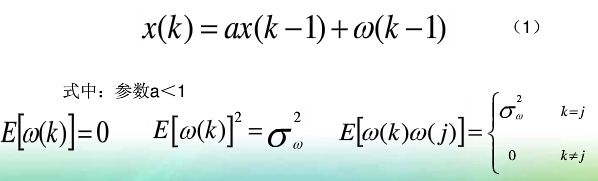信号测量过程的数学模型为：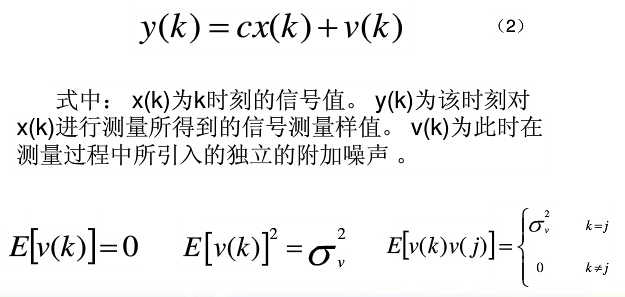所以可以得到一维时变随机信号及其测量过程的数学模型。如下所示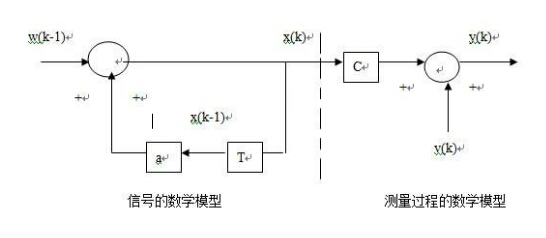一维随机信号的递归型估计其的一般表达式：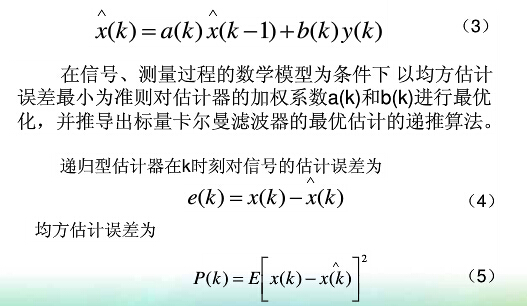代入递归型估计器的一般表达式，得：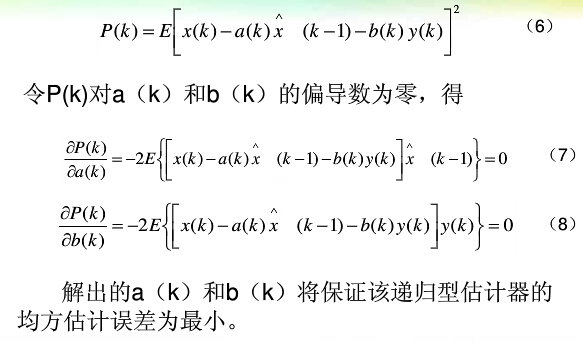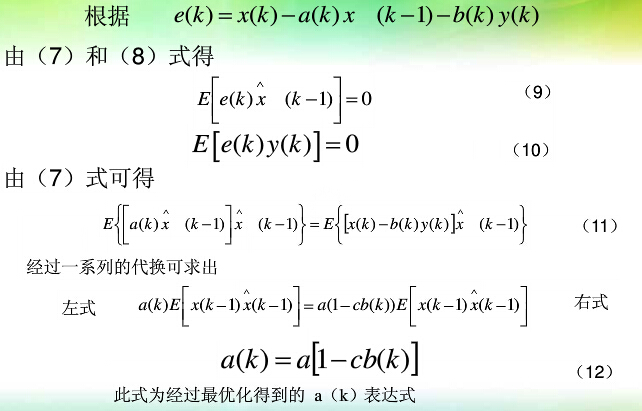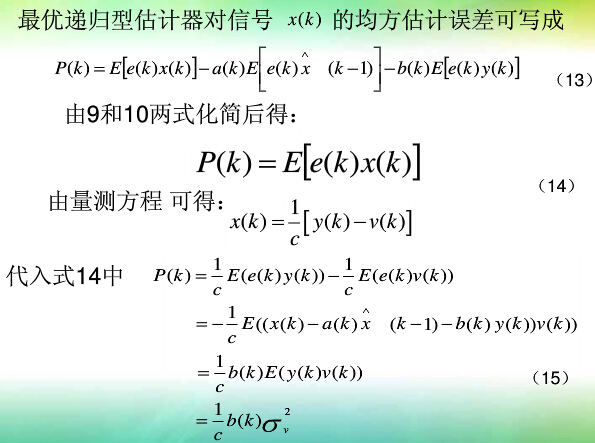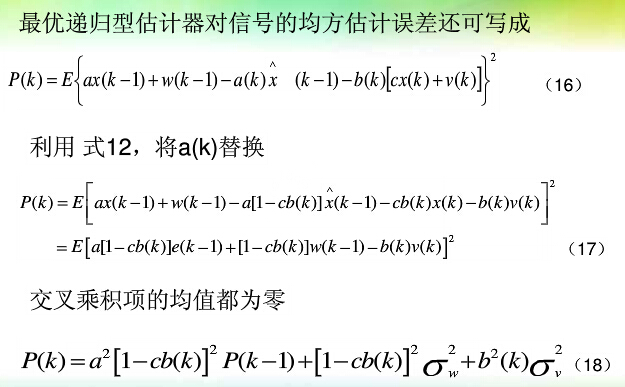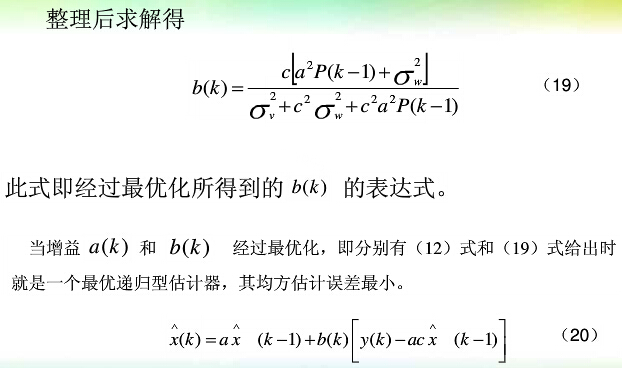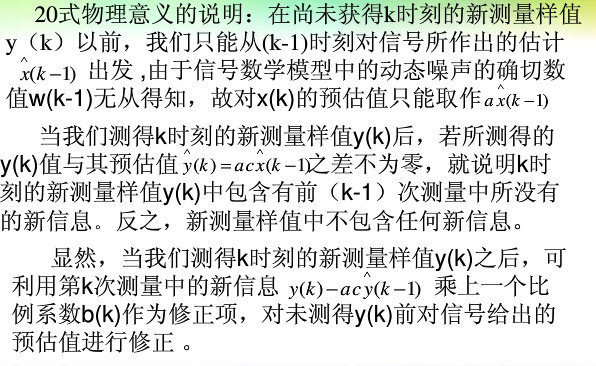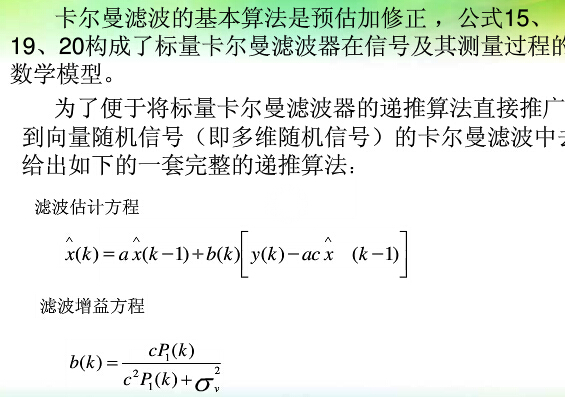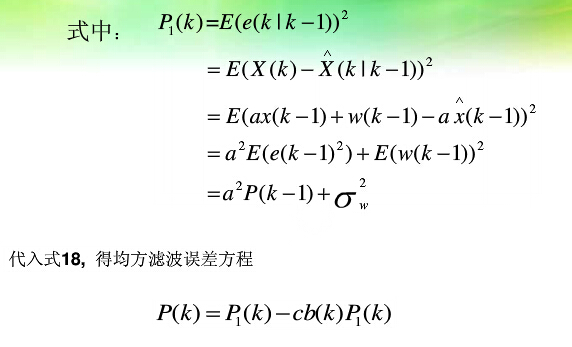在实际应用中，常用到向量卡尔曼滤波算法，因此需要一些转化。该转化主要为标量运算和矩阵运算的差异：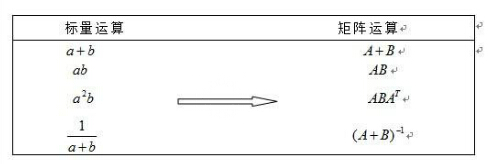修正差异后，可以直接将标量KF推广到向量KF。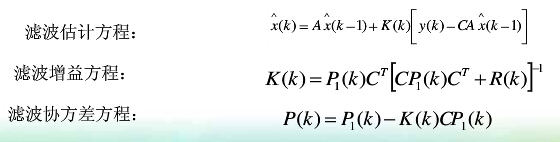2. 扩展卡尔曼滤波学习
由于卡尔曼滤波估计是一个线性随机系统的状态。然而实际中，很多系统是非线性的，处理这些系统时，用扩展卡尔曼滤波（EKF），它是将期望和方差线性化的卡尔曼滤波器。3. 扩展卡尔曼滤波的matlab实现
下面这段代码应用于空中飞鼠的数据处理中。
q为四元数，wb为角度偏置，z，h，y均为中间向量，a为加速度计原数据，w为陀螺仪原数据，dt为积分时间
function [ q, wb,z,h,y] = ekf2( a,w,dt )
persistent x P;
% Q
Q=zeros(6,6);
Qwb=.01;
Q(5,5)=Qwb;
Q(6,6)=Qwb;
% R, DMP take 1s converge,
R=eye(3)*1e4;
% P
if isempty(P)
P = eye(length(Q))*1e4;
x = [1, 0, 0, 0, 0, 0]';
end
%%%%%%%%% inputs %%%%%%%%%
%save x_k-1
q0 = x(1);q1 = x(2);q2 = x(3);q3 = x(4);
wxb= x(5);  %bias
wyb= x(6);
%input
wx = w(1);
wy = w(2);
wz = w(3);
z=a';
%%%%%%%%%%%%%%%%%%
% Populate F jacobian= d(f)/d(x_k-1/k-1)
F = [              1, -(dt/2)*(wx-wxb), -(dt/2)*(wy-wyb), -(dt/2)*(wz),  (dt/2)*q1,  (dt/2)*q2;
(dt/2)*(wx-wxb),                1,  (dt/2)*(wz), -(dt/2)*(wy-wyb), -(dt/2)*q0,  (dt/2)*q3;
(dt/2)*(wy-wyb), -(dt/2)*(wz),                1,  (dt/2)*(wx-wxb), -(dt/2)*q3, -(dt/2)*q0;
(dt/2)*(wz),  (dt/2)*(wy-wyb), -(dt/2)*(wx-wxb),                1,  (dt/2)*q2, -(dt/2)*q1;
0,                0,                0,            0,          1,          0;
0,                0,                0,            0,          0,          1;];
%%%%%%%%% PREDICT %%%%%%%%%
%Predicted state estimate
% x_k/k-1 = f(x_k-1/k-1,u_k-1)
% refer "Appling", p17, x_t+1=x_t+g(x_t)*ts
x = [q0 + (dt/2) * (-q1*(wx-wxb) - q2*(wy-wyb) - q3*(wz));
q1 + (dt/2) * ( q0*(wx-wxb) - q3*(wy-wyb) + q2*(wz));
q2 + (dt/2) * ( q3*(wx-wxb) + q0*(wy-wyb) - q1*(wz));
q3 + (dt/2) * (-q2*(wx-wxb) + q1*(wy-wyb) + q0*(wz));
wxb;
wyb;];
%
% Normalize Quaternion
x(1:4)=x(1:4)/norm(x(1:4));
q0 = x(1);q1 = x(2);q2 = x(3);q3 = x(4);
% Predicted covariance estimate, P_k/k-1=F_k-1*P_k-1/k-1*F_k-1'+Q_k-1
P = F*P*F' + Q;
%%%%%%%%%% UPDATE %%%%%%%%%%%
%%% h(x_k/k-1)
h = [2*q1*q3 - 2*q0*q2; % refer AN4676, page 26
2*q0*q1 + 2*q2*q3;
q0^2 - q1^2 - q2^2 + q3^2;];
%Measurement residual
% y_k = z_k - h(x_k/k-1), where h(x) is the matrix that maps the state onto the measurement
% z_k: Acc, h=[0 0 g/g]*[quaternion-> rotation_matrix ]
% y(3x1)=z(3x1)-h(3x1)
y = z - h;
%%%%%%%%%%%%%%%%%%
% The H matrix maps the measurement to the states
% H=d(h)/d(x_k/k-1)
H = [-2*q2,  2*q3, -2*q0,  2*q1, 0, 0;
2*q1,  2*q0,  2*q3,  2*q2, 0, 0;
2*q0, -2*q1, -2*q2,  2*q3, 0, 0;];
%%%%%%%%%%%%%%%%%%
% Measurement covariance update,S_k=H_k*P_k/k-1*H_k'+R_k
S = H*P*H' + R;
%%%%%%%%%%%%%%%%%%
% Calculate Kalman gain,K_k=P_k/k-1*H_k'*S_k^-1
% K(7x3) = P(7x7)*H'(7x3)/S(3x3);
K = P*H'/S;
%%%%%%%%%%%%%%%%%%
% Corrected model prediction, x_k/k=x_k/k-1+K_k*y_k
% x(7x1) = x(7x1) + K(7x3)*y(3x1)
x = x + K*y;      % Output state vector
%
%%%%%%%%%%%%%%%%%%
% Update state covariance with new knowledge,P_k/k=(I-K_k*H_k)*P_k/k-1
I = eye(length(P));
P = (I - K*H)*P;  % Output state covariance
%%%%%%%%%%%%%%%%%%
q = [x(1), x(2), x(3), x(4)];
wb = [x(5), x(6)];
end

展开全文matlab
一、引言

本文工分为以下几个部分：

1、引言

2、模型的系统方程和状态方程

3、卡尔曼滤波过程及五个基本公式

4、公式中每个参数详细注释

6、MATLAB实现滤波器

二、模型的系统方程和状态方程

系统的状态方程：

状态方程是根据上一时刻的状态和控制变量来推测当前时刻的状态，是服从高斯分布的噪声，是预测过程的噪声，它对应了  中每个分量的噪声，是期望为 0，协方差为 Q 的高斯白噪声,Q即下文的过程激励噪声Q.

系统的观测方程：

观测方式是当前时刻的量测信息，是观测的噪声，服从高斯分布，,R即下文的测量噪声R。

卡尔曼滤波算法有两个基本假设:
( 1) 信息过程的足够精确的模型，是由白噪声所激发的线性( 也可以是时变的) 动态系统;

( 2) 每次的测量信号都包含着附加的白噪声分量 。当满足以上假设时，可以应用卡尔曼滤波算法。

三、 卡尔曼滤波过程及五个基本公式

卡尔曼滤波时间更新（预测）
1. 向前推算状态变量

2. 向前推算误差协方差

卡尔曼滤波测量更新（校正）

3. 计算卡尔曼增益

4. 由观测变量更新估计

5. 更新测量误差

四、 公式中每个参数详细注释

和：   分别表示 时刻和 时刻的后验状态估计值，是滤波的结果之一，即更新后的结果，也叫最优估计（估计的状态，根据理论，我们不可能知道每时刻状态的确切结果所以叫估计）。

：    时刻的先验状态估计值，是滤波的中间计算结果，即根据上一时刻（时刻）的最优估计预测的时刻的结果，是预测方程的结果。

和 ：  分别表示 k － 1 时刻和 k 时刻的后验估计协方差（即 和  的协方差，表示状态的不确定度），是滤波的结果之一。

：  k 时刻的先验估计协方差（的协方差），是滤波的中间计算结果。

： 是状态变量到测量（观测）的转换矩阵，表示将状态和观测连接起来的关系，卡尔曼滤波里为线性关系，它负责将 m 维的测量值转换到 n 维，使之符合状态变量的数学形式，是滤波的前提条件之一。

： 测量值（观测值），是滤波的输入。

： 滤波增益矩阵，是滤波的中间计算结果，卡尔曼增益，或卡尔曼系数。

： 状态转移矩阵，实际上是对目标状态转换的一种猜想模型。例如在机动目标跟踪中， 状态转移矩阵常常用来对目标的运动建模，其模型可能为匀速直线运动或者匀加速运动。当状态转移矩阵不符合目标的状态转换模型时，滤波会很快发散。

： 过程激励噪声协方差（系统过程的协方差）。该参数被用来表示状态转换矩阵与实际过程之间的误差。因为我们无法直接观测到过程信号， 所以 Q 的取值是很难确定的。是卡尔曼滤波器用于估计离散时间过程的状态变量，也叫预测模型本身带来的噪声。状态转移协方差矩阵。

： 测量噪声协方差。滤波器实际实现时，测量噪声协方差 R一般可以观测得到，是滤波器的已知条件。

： 是将输入转换为状态的矩阵。

： 实际观测和预测观测的残差，和卡尔曼增益一起修正先验（预测），得到后验。

1、 建立模型系统方程和量测方程

可得：A 为，B为，为高斯白噪声可不关心

2、建立量测方程

可得：H为，为量测噪声可不关心

3、分析Q和R
假如已经分析出一系列的数据，则;

假如已经分析出一些列的数据，则;

4、初始值确定
给滤波过程的初始状态初始化。

5、最后一步，就是对照公式根据理解，套公式，写程序。

六、MATLAB实现滤波器

1、 滤波器设计
function z = kalmanFilter(x)
%%%%%%%%%%%%%%%%%%%%%%%%%%%%%%%%%%%%%%%%%%%%%%%%%%%%%%%%%%%%%%%%%%%%%%%%%%%
%
%  function z = kalmanFilter(x)
%
%>
%> @brief 一维卡尔曼滤波
%>
%> @param[out]  z             滤波后的结果
%> @param[in]   x             需要滤波的数据
%>
%
%%%%%%%%%%%%%%%%%%%%%%%%%%%%%%%%%%%%%%%%%%%%%%%%%%%%%%%%%%%%%%%%%%%%%%%%%%%

% 卡尔曼相关变量定义
persistent xk xk_1;             % 状态量
persistent zk;                  % 观测量
persistent A;                   % 状态转移矩阵
%     persistent B;                   % 控制输入模型
persistent H;                   % 观测矩阵
persistent Pk Pk_1;             % 误差协方差矩阵
persistent Q;                   % 状态噪声协方差矩阵
persistent R;                   % 观测噪声协方差矩阵

% 卡尔曼相关参数初始化
if isempty(xk)
A = 1;
H = 1;
Pk = 1;
Pk_1 = 1;                   % 初始误差协方差为1
xk = 0;
xk_1 = 0;
zk = 0;
end

I = 1;
if xk_1 == 0
xk_1 = x;
xk = x;
else
zk = H*x;                   % 观测量方程
% 预测
X = A*xk_1;                 % 状态预测
P = A*Pk_1*A' + Q;          % 误差协方差预测
% 更新(校正)
K = P*H'*inv(H*P*H'+R);     % 卡尔曼增益更新
xk = X + K*(zk - H*X);      % 更新校正
xk_1 = xk;                  % 保存校正后的值，下一次滤波使用
Pk = (I - K*H)*P;           % 更新误差协方差
Pk_1 = Pk;                  % 保存校正后的误差协方差，下一次滤波使用
end

% 滤波结果返回
z = xk;
end

2、仿真运行
%% 1. 导入数据
[fname, pname] = uigetfile('*', 'Sample Dialog Box');
fileID = fopen(strcat(pname, fname));

%% 2. 卡尔曼滤波
end

%% 3. 滤波检验
figure(1);
plot(rssi, 'Color', 'r', 'Marker', 'o'); hold on;
plot(rssi_opt, 'Color', 'b', 'LineStyle', '-', 'Marker', '+'); hold off;
title('rssi滤波对比');

3、运行结果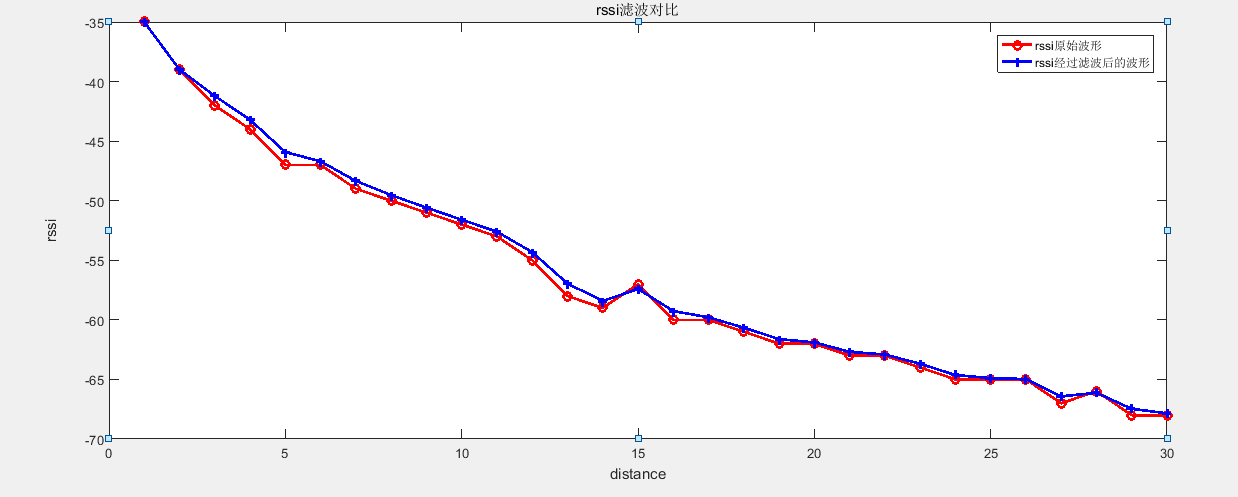展开全文• 扩展卡尔曼滤波_无迹卡尔曼滤波_扩展信息滤波_l粒子滤波算法.rar
• ## 卡尔曼滤波算法

千次阅读 2017-11-28 21:32:45
Kalman Filter是一个高效的递归...本文介绍了卡尔曼滤波增益的由来，以及它在卡尔曼滤波理论中的作用，着重介绍了卡尔曼滤波增益的理论意义。由卡尔曼滤波增益可以更深入的理解卡尔曼滤波，把它更好地应用于实际中。
• 卡尔曼滤波（Kalman Filter）是一种利用线性系统状态方程，利用对系统的观测数据，对系统状态进行最优估计的算法。由于观测数据受到系统中的噪声和干扰的影响，所以系统状态的估计过程也可看作是滤波过程。应用场景...信号处理 自适应卡尔曼
• 所有滤波问题其实都是求感兴趣的状态的后验概率分布，只是由于针对特定条件的不同，可通过求解析解获得后验概率（KF、EKF、UKF），也可通过大数统计平均求期望的方法来获得后验概率（粒子滤波）。 KF、EKF、UKF ...
• 卡尔曼滤波算法是一种基于最小均方误差的最优线性递归滤波方法，以状态方程和观测方程为基础，运用递归方法来预测线性系统变化。 状态方程和观测方程如下： X(k)是k时刻的状态向量，A是状态系统矩阵，是状态系统...运动目标检测
• matlab卡尔曼滤波和扩展卡尔曼滤波（KF&EKF）例程，可供学习参考使用，谢谢！ 希望能帮到你。。。。。。。。。。。
• 卡尔曼滤波理论及应用-卡尔曼滤波与维纳滤波（哈工大）.part1.rar 卡尔曼滤波理论及应用 Unnamed QQ Screenshot20121023091849.png 卡尔曼滤波与维纳滤波（哈工大）.part...matlab
• 卡尔曼滤波理论及应用-卡尔曼滤波与维纳滤波（哈工大）.part2.rar 卡尔曼滤波理论及应用 Unnamed QQ Screenshot20121023091849.png 卡尔曼滤波与维纳滤波（哈工大）.part...matlab
• 卡尔曼滤波理论及应用-卡尔曼滤波与维纳滤波（哈工大）.part3.rar 卡尔曼滤波理论及应用 Unnamed QQ Screenshot20121023091849.png 卡尔曼滤波与维纳滤波（哈工大）.part...matlab
• 传统的卡尔曼滤波算法假定了噪声服从高斯分布，而实际应用场景中，由于传感器受到各种因素的影响，可能存在部分远偏离预期值的观测结果，称为奇异值。此时观测噪声不再是高斯分布的，而类似于student-t分布，对于...
• 在信息融合中经常使用卡尔曼滤波，现在我们对其进行讲解： 百度百科上写到： 卡尔曼滤波公式如上 这是另一种表述，涉及的符号见下表： 对于联邦卡尔曼滤波： 对于一致性卡尔曼滤波： ...信号处理 传感器
• 卡尔曼滤波理论与实践(MATLAB版)(第四版) 莫欣德 S.格雷沃 (Mohinder S.Grewal) (作者), 安格斯 P.安德鲁斯 (Angus P.Andrews) matlab代码，卡尔曼滤波各种实例分析
• ## 详解卡尔曼滤波原理

万次阅读 多人点赞 2017-03-18 13:54:15
详解卡尔曼滤波原理　在网上看了不少与卡尔曼滤波相关的博客、论文，要么是只谈理论、缺乏感性，或者有感性认识，缺乏理论推导。能兼顾二者的少之又少，直到我看到了国外的一篇博文，真的惊艳到我了，不得不佩服作者...
• 卡尔曼滤波理论及应用-基于扩展卡尔曼滤波的汽车质心侧偏角估计.pdf 卡尔曼滤波理论及应用 Unnamed QQ Screenshot20121023091849.png 卡尔曼滤波与维纳滤波（哈工大）....matlab